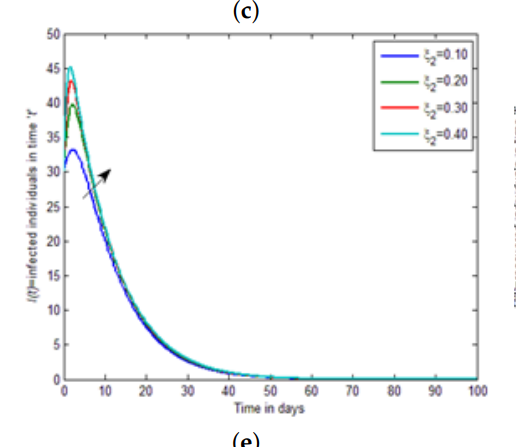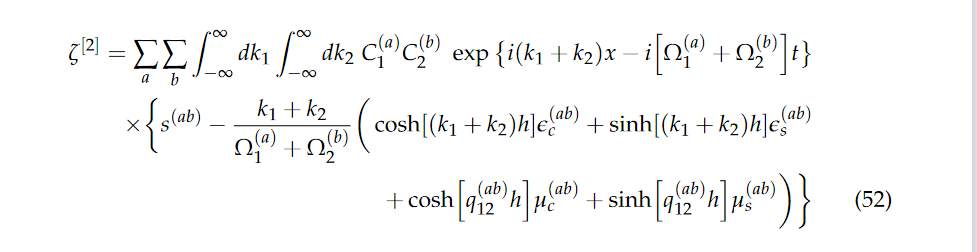## Neutral DDEs with 'consistent' initial conditions?...

I'd like to reproduce Initial Value DDE of Neutral Type in Maple.
The differential equation is:

`deq := D(y)(t) = 2*cos(2*t)*y(t/2)^(2*cos(t)) + ln(D(y)(t/2)) - ln(2*cos(t)) - sin(t): # with y(0) = 1 and known D(y)(0)`

Unfortunately, if I type valid initial values, Maple will simply generate , and yet if I just give a partial initial condition, Maple will display and only return incorrect results.

 > restart;
 >(1)
 >>(2)
 >>>>The output is wrong. Note that "y(0) = 1" is insufficient to uniquely specify a solution, as "D(y)(0)" can be either -LambertW(-2/exp(2)) or 2. But Maple does not allow sufficient constraints here. How do I avoid such an unexpected behavior?

## Use of 2-D FFT of 'large' matrices: inefficient?!...

Here is a test:For small matrices, apart from the first call, the performance is almost perfect (🎉!).
As a comparison, an equivalent test may be performed in modern Python:As you can see, for 1024×1024, 2048×2048, 4096×4096, 8192×8192, and 16384×16384 matrices, Maple's performance gets pretty poor. Is the `FFT` procedure not well optimized for larger matrices? I do have read the Fourier Transforms in Maple, yet I cannot find any information on this subject.
In accordance with the following output

```showstat(DiscreteTransforms::FFT_complex8, 3):

FFT_complex8 := proc()
...
...
end proc
```

it appears that the code hasn't been developed for 20 years. Is it possible to improve the performance of the `FFT` built into Maple in order that the computation on such a 2¹⁴×2¹⁴ matrix can be achieved in about twenty seconds (rather than in two minutes)?

Note. For these matrices, exact transform results (see below) can be obtained symbolically.

```for n from 0 to 12 do
m := LinearAlgebra:-HankelMatrix(<\$ (1 + 1 .. 2**n + 2**n)>, datatype = complex, shape = []): gc();
print(n, andseq(abs(_) < HFloat(1, -10, 2), _ in SignalProcessing:-FFT(m, normalization = none, inplace = true) - Matrix(2^n, <2^(2*n)*(2^n + 1), <'2^(2*n - 1)*(:-cot((k - 1)/2^n*Pi)*I - 1)' \$ 'k' = 1 + 1 .. 2^n>>, shape = symmetric, storage = sparse, datatype = complex)) (* faster than `rtable_scanblock` and `ArrayTools:-IsZero` and much faster (🎊!) than `comparray` and `verify/Matrix` with testfloat *) )
od:
=
0, true

1, true

2, true

3, true

4, true

5, true

6, true

7, true

8, true

9, true

10, true

11, true

12, true

```

However, the main goal is to test the numerical efficiency of Maple's fast Fourier transform algorithm.

## Typesetting: some equestions on argument delimiter...

Q1: Why does Maple use the bar as a delimiter for certain elliptic expressions and the comma for others?

Is that in line with: Gradshteyn and Ryzhik (G&R) and in the popular "Handbook of Mathematical Functions" edited by Abramowitz and Stegun (A&S), as stated in help(JacobiAM)?

Q2: Can I have the comma instead of the vertical bar for the Jacobian functions?

Q3: If not, how to get a bit more space between the symbols and the bar for better readability?(1)(2)(3)(4)## Crank–Nicolson Finite difference scheme ...

How to get same graph from maple with finite difference method for differential equations

I m new here how to plot this i have seen related posts no where given clear idea for FDM method

plase help me to get the results Thank you## How can I get the Compiler to work?...

I made the upgrade to Maple 2023 today and for fun I compiled a simple procedure. I got an error in Maple 2023. So I ran the same lines of code in Maple 2022 and eveything works. Does anyone sees this problem?

 > restart: kernelopts(version);(1)
 > p := proc( x :: float ) :: float; 2.3 * x end proc:
 > cp:=Compiler:-Compile(p)
 > cp(1.1)(2)
 >

Suppose that a procedure is declared with option threadsafe and it has a local child procedure PC (possibly anonymous). Is their any benefit, or perhaps any detriment, to also declaring PC with option threadsafe? For example, is there any benefit or detriment to the yellow option threadsafe in this code below?:

P:= proc()
local PC:= proc()
option threadsafe; (* some code *) end proc;
(* some code *)
PC();
(* some code *)

end proc;

## Bifurcation Diagram from my system of equations...

 >(1)
 >(2)(3)(4)(5)(6)(7)(8)(9)(10)
 >>(11)
 >>>(12)
 >(13)
 >(14)
 >(15)
 >(16)
 >(17)
 >(18)
 >(19)
 >(20)
 >(21)
 >(22)
 >(23)
 >(24)
 >(25)
 >(26)
 >(27)
 >(28)
 >(29)
 >(30)
 >(31)
 >(32)

## how do I want to produce a graph that has differen...the graf that I want to generate is like this one

## numerical output and plot integral...

Dear experts

how can I numerically plot the following integral and have output as csv file.

in this relation, there is a list of omega1 and omega2 for each k1 and k2. for example,

k1 = [1,2,3,4,5]

omega11=[1,2,3,4,5], omega12= [1,2,3,4,5], omgega21= [1,2,3,4,5],omega22= [1,2,3,4,5],

all other coefficients would be calculated based on k values and corresponding omegas## Solving pdes with multiple functions....

Given a pde (or a set of pdes) of multiple funcitons, is there a way to look for solutions when one of the functions is kept arbritary.

For example if I have a set of pdes with f1,f2,f3. Is there a way to see if there is a functional form for f2 and f3 such that the equation is satisfied for any f1?

## with respect to side relations or using the assume...

It appears to me that "simplify/siderels" with two arguments is simply some special "simplify" procedure that just makes use of assumptions, but the results of experiments seem to tell an opposite story.

```simplify(sqrt(x**2), [x = 0]);
0

simplify(sqrt(x**2), assume = [x = 0]);
0

simplify(ln(exp(x)), [x = 0]);
0

simplify(ln(exp(x)), assume = [x = 0]);
x

`assuming`(simplify(ln(exp(x))), [x = 0]);
x

simplify((1 - cos(x)**2 + sin(x)*cos(x))/(sin(x)*cos(x) + cos(x)**2), [sin(x)**2 + cos(x)**2 = 1]);
2
1 - cos(x)  + sin(x) cos(x)
---------------------------
cos(x) (cos(x) + sin(x))

simplify((1 - cos(x)**2 + sin(x)*cos(x))/(sin(x)*cos(x) + cos(x)**2), assume = [sin(x)**2 + cos(x)**2 = 1]);
tan(x)

```

How to explain these?

## Problem entering a math equation in a text region....

Today, I trried to enter an equation into a text region using Ctrl+R (In Maple Flow 2023.1), like I have always done in previous releases of Maple Flow. Well, today it did not work. I tried to enter the equation Q=W+mCv(T2-T1). When I tried to enter the equal sign after the Q, the program would not allow me to do it. I would press the equal sign and nothing happened. I tried :=, but it would only enter the :, it would not let me enter the =. Any help will be appreciated.

## How to define properly multi-variate statistical d...

(While using Maple 2015 this question concerns any other Maple versions)

I hesitated on the title to write and my first idea was to write "How to modify a built-in functions without making a mess?".
I finally changed my mind in order not to orient the answers in a wrong way.

So this question is about the construction of multi-variate distributions and concerns only the Statistics package.
Here are some of the attributes of a univariate random variable that Maple recognizes, and it is quite normal to expect that the construction of a multi-variate random variable (MVRV for short) distribution should get, at least, some of them.

```X := RandomVariable(Normal(a, b)):
map(a -> printf("%a\n", a), [exports(attributes(X))]):
Conditions
ParentName
Parameters
CharacteristicFunction
CDF
CGF
HodgesLehmann
Mean
Median
MGF
Mode
PDF
RousseeuwCrouxSn
StandardDeviation
Support
Variance
CDFNumeric
QuantileNumeric
RandomSample
RandomSampleSetup
RandomVariate
MaximumLikelihoodEstimate
```

If the distribution is continuous the PDF is fundamental in the sense it enables constructs all the other statistics (=attributes) of a MVRV.
But it is nice to use integrated functions, such as Mean, Support, PDF, and so on, to get the expressions or values of these statistics instead of computing them from this PDF.
Let's that I prefer doing this

```MyNormal := proc(m, v)
description "Reparameterized Normal randomvariable, m=mean, v=variance":
Distribution(
PDF = (t -> exp(-1/2*(x-m)^2/v)/sqrt(2*Pi*v))
, Conditions = [Sigma > 0]
, Mean =m
)
end proc:

X := RandomVariable(MyNormal(mu, Sigma)):
Mean(X)
m```

than doing this

```MyNormal := proc(m, v)
description "Reparameterized Normal randomvariable, m=mean, v=variance":
Distribution(
PDF = (t -> exp(-1/2*(x-m)^2/v)/sqrt(2*Pi*v))
, Conditions = [Sigma > 0]
)
end proc:

X := RandomVariable(MyNormal(mu, Sigma)):
Mean(X);  # of course undefined
mean := int(PDF(X, x), x=-infinity..+infinity) assuming Sigma > 0
undefined
mean := 1
```

So, while all the statistics can be recover from the CDF (provided it exists), it's nicer to define these statistics within the Distribution structure (as in the first construction above).

Now some problems appear when you want to construct the Distribution structure for a MVRV.
The attached file contains the construction of MVRV whose ecah components are mutually independent (to keep the things simple) and both have a Unifom distribution.

MV_Uniform.mw

Here are some observations:

• Defining a multi-variate PDF goes without problems.
• Defining the Mean (or many other algebraic or numeric statistics) presents a difficulty related to the type of the arguments the build-in function Mean is aimed to recieve.
But a workaround, not very elegant, can be found.
• The case of the Support seems unsolvable: I wasn't able to find any workaround to define the support of a MVRV.
• I did not consider the Conditions attribute, but I'm not sure that, in the case of, let's say, a bi-gaussian random variable I would be capable to set that the variance is a symmetric positive-definite matrix?

I feel like the main restriction to define such MVRV distributions is the types used in the buid-in functions used in the Distribution structure.

Does anyone have an idea to tackle this problem?

• Are we doomed to use workarounds like the one I used for defining the MVRV mean?
• Can we modify the calling sequence of some build-in functions without making a mess and keep them working on build-in distributions?
• Must we overload the construction of these build-in functions?
Doing for instance:
```restart:
with(Statistics):
local Mean:
Mean := proc(...) ... end proc```

Thanks in advance for any suggestion and help.

## about root decomposition in Lie algebras...

For the command LieAlgebras[RootSpaceDecomposition] I don't understand what the command return, I read the help and see the examples but still not understanding.

for example it returns:

RSD := RootSpaceDecomposition(CSA);

RSD := table([[-2, -1] = E31, [2, 1] = E13, [1, 2] = E23, [1, -1] = E12, [-1, 1] = E21, [-1, -2] = E32])

I don't understand what means [-2, -1] even they said that is the root but I know that a root is in h* so it must be only a number not a vector.